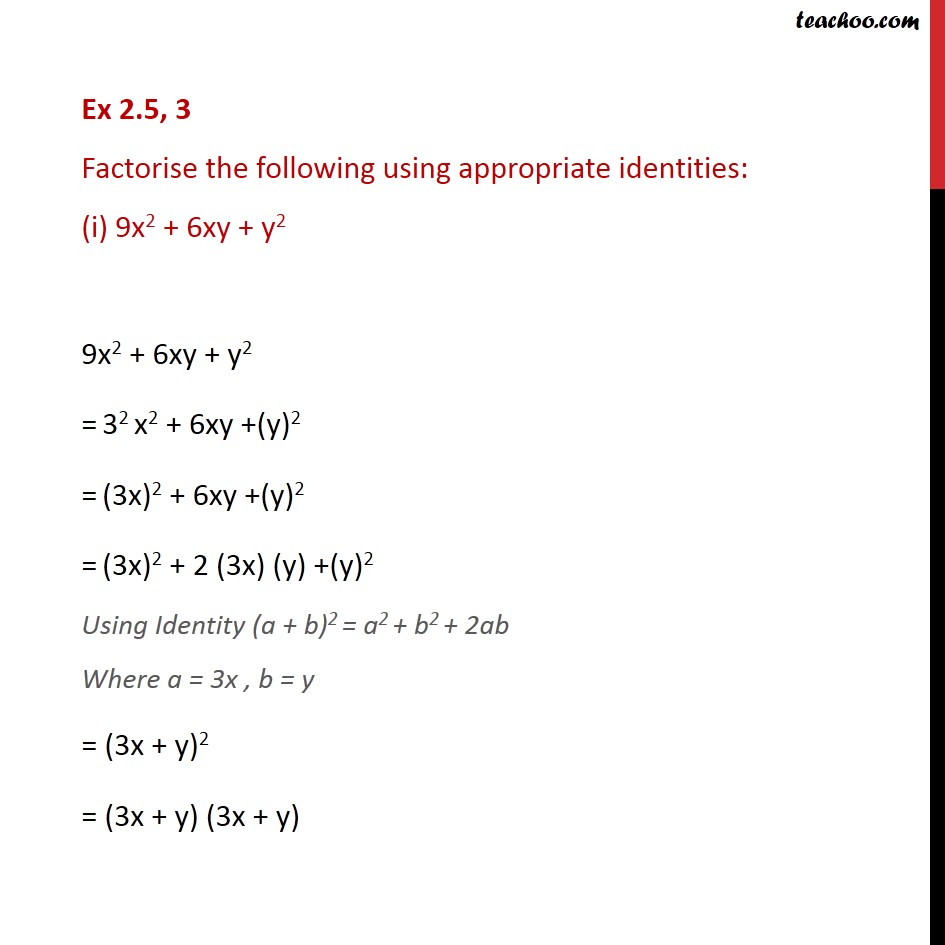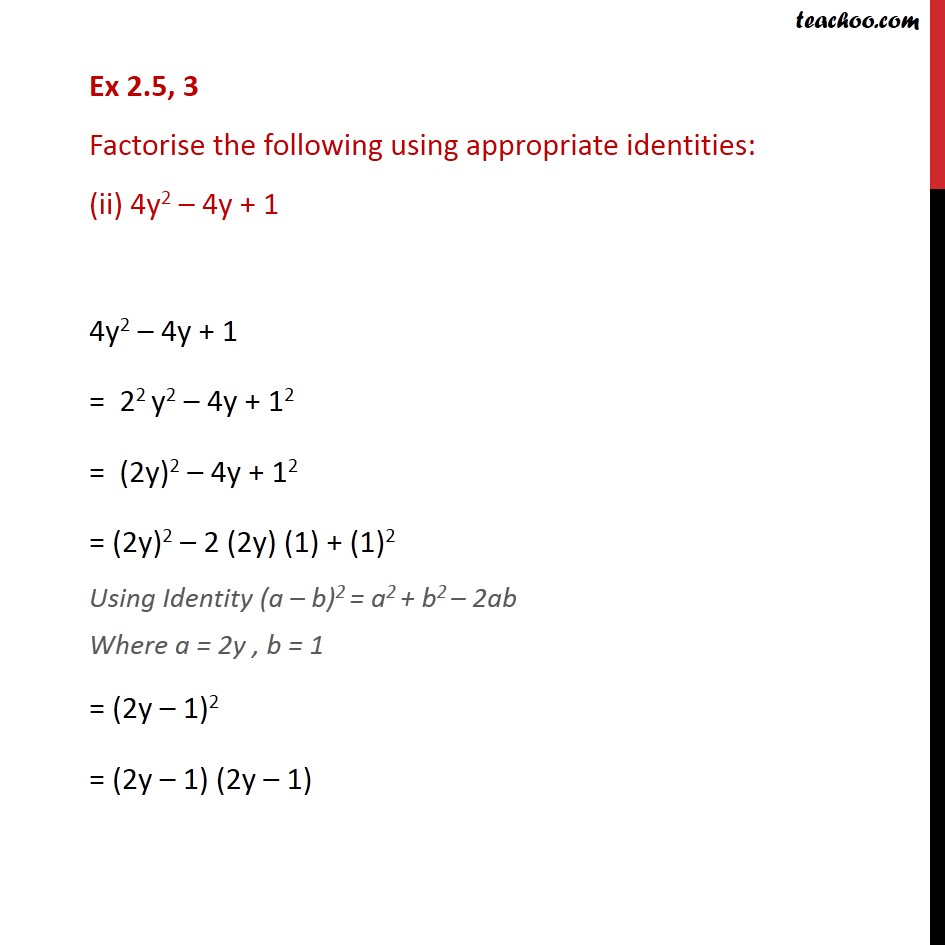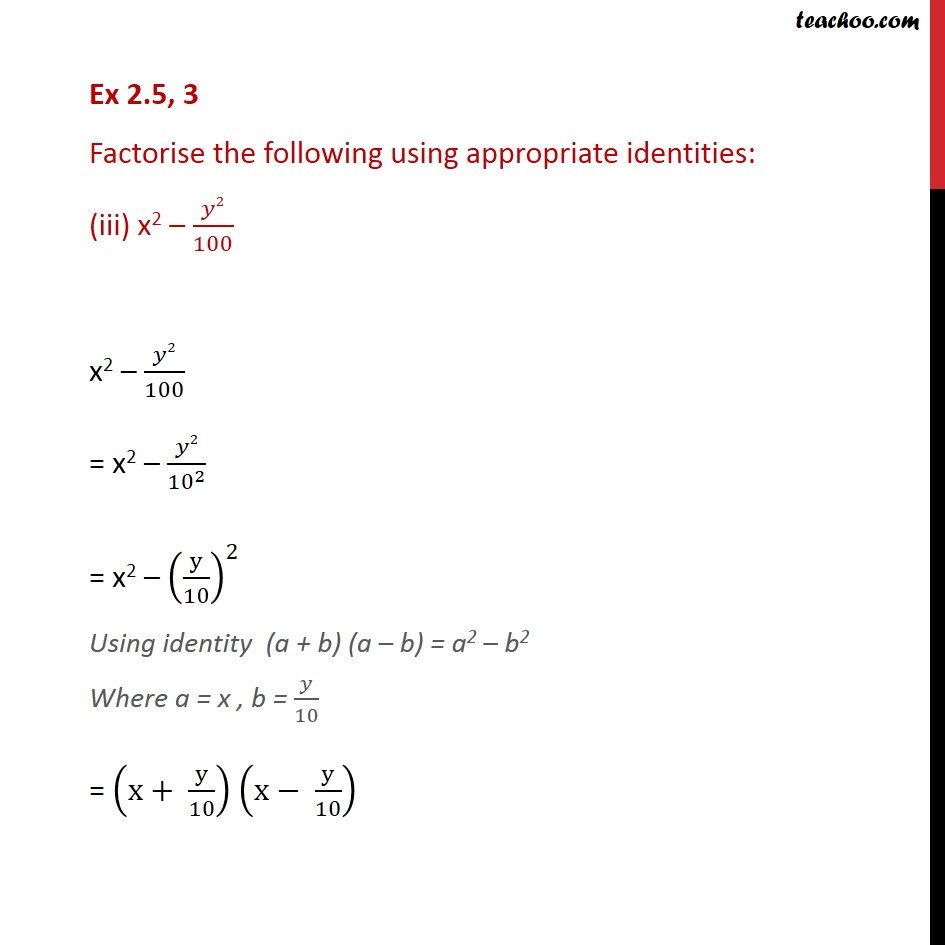1. Chapter 2 Class 9 Polynomials
2. Serial order wise
3. Ex 2.5

Transcript

Ex 2.5, 2 Evaluate the following products without multiplying directly: (iii) 104 96 104 96 = (100 + 4) (100 4) Using the identity (x + y) (x y) = x2 y2 where x = 100 , y = 4 = (100)2 (4)2 = 10000 16 = 9984 Ex 2.5, 3 Factorise the following using appropriate identities: (i) 9x2 + 6xy + y2 9x2 + 6xy + y2 = 32 x2 + 6xy +(y)2 = (3x)2 + 6xy +(y)2 = (3x)2 + 2 (3x) (y) +(y)2 Using Identity (a + b)2 = a2 + b2 + 2ab Where a = 3x , b = y = (3x + y)2 = (3x + y) (3x + y) Ex 2.5, 3 Factorise the following using appropriate identities: (ii) 4y2 4y + 1 4y2 4y + 1 = 22 y2 4y + 12 = (2y)2 4y + 12 = (2y)2 2 (2y) (1) + (1)2 Using Identity (a b)2 = a2 + b2 2ab Where a = 2y , b = 1 = (2y 1)2 = (2y 1) (2y 1) Ex 2.5, 3 Factorise the following using appropriate identities: (iii) x2 2/100 x2 2/100 = x2 2/ 10 ^2 = x2 (y/10)^2 Using identity (a + b) (a b) = a2 b2 Where a = x , b = /10 = (x+ y/10) (x y/10)

Ex 2.5

Chapter 2 Class 9 Polynomials
Serial order wise